# Diagonal of a Square

Back to “Geometry” Page

The diagonal of a square is as simple as the Pythagorean theorem.

It is very simple:

(length)2 + (width)2 = (diagonal)2

In other words, you simply take the square root of the sum of the squares of the length and width of the square.

(length)2 + (width)2   = d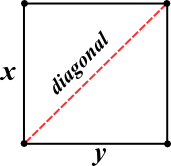A simple equation for the diagram above would be this:

x2 + y2   = d

Since the length and width of a square are equal, you can simply multiply one side squared by 2, like this:

2(x2) = d

So, if a side of the square above is equal to 1, then the equation for the diagonal is this:

2(12) = d

2  = d

Incidentally,  2  is approximately 1.4. That’s a handy number to remember.

And, if a side of the square above is equal to 2, then the equation for the diagonal is this:

2(22) = d

2(4)  = d

2 2  = d

And, if a side of the square above is equal to 3, then the equation for the diagonal is this:

2(32) = d

2(9)  = d

3 2  = d

You can now see a pattern developing. The diagonal of a square is equal to any side of the square times  2 .

See whether you can solve the flashcards below.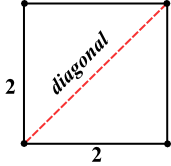• What is the diagonal of the square above?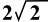• What is the area of the square above?
4
• What is the perimeter of the square above?
8
• What's the area of the isoceles triangle?
2• What is the diagonal of the square above?• What is the area of the square above?
25
• What is the perimeter of the square above?
20
• What's the area of the isoceles triangle?
12 1/2• What is the diagonal of the square above?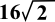• What is the area of the square above?
256
• What is the perimeter of the square above?
64
• What's the area of the isoceles triangle?
128Assume that the circle touches all four sides of the square in the diagram above. Remember that you may give answers for area and circumference of a circle in terms of π, as in 2π or 7π and so on.

• What is the diameter of the circle above?
6
• What is the area of the square above?
36
• What is the perimeter of the square above?
24
• What's the circumference of the circle?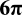• What's the area of the circle?